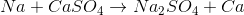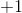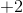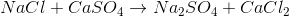# High School Chemistry : Help with Single-Replacement Reactions

## Example Questions

### Example Question #2 : Oxidation Reduction Fundamentals

Which of the following reactions involves both reduction and oxidation reactions?

Possible Answers:

Single displacement reactions

Neither single displacement reactions nor the reaction of sodium chloride and calcium sulfate

Both single displacement reactions and the reaction of sodium chloride and calcium sulfate

The reaction of sodium chloride and calcium sulfate

Correct answer:

Single displacement reactions

Explanation:

A reaction that has both reduction and oxidation half reaction is called a redox reaction. It involves one or more atoms gaining electrons (reduction) and one or more atoms losing electrons (oxidation). Recall that single displacement reactions involve the replacement of an element in a compound with another element. An example of single replacement reaction is shown below:In this reaction, a sodium atom replaces a calcium atom in calcium sulfate. If we calculate the oxidation numbers for sodium and calcium, we can see that sodium loses an electron (the oxidation state goes fromto) whereas the calcium ion gains two electrons (goes fromto); therefore, this is a redox reaction. All single displacement reactions follow this general trend and are characterized as redox reactions.

Reaction of sodium chloride and calcium sulfate is as follows:If we calculate the oxidation state of each atom we will notice that oxidation number doesn’t change; therefore, this isn’t a redox reaction.

### All High School Chemistry Resources